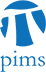## DG-MP-PDE Seminar: Volume Growth, Brownian motion, and Conservation of the heat kernel on a Riemannian manifold

• Date: 10/06/2009
Lecturer(s):

Elton Hsu (Northwestern University)

Location:

University of British Columbia

Description:

Abstract: The minimal heat kernel on a Riemannian manifold is conservative if it integrates to 1. If this is the case, the manifold is said to be stochastically complete. Since the heat kernel is the transition density function of Brownian motion, a manifold is stochastically complete if and only if Brownian motion does not explode. This interpretation opens a way for investigating conservation of the heat kernel by probability theory. To find a proper geometric condition for heat kernel conservation is an old geometric problem. The first result in this direction was due to S. T. Yau, who proved that a Riemannian manifold is stochastically complete if its Ricci curvature is bounded from below by a constant. However, it has been known for quite some time that the heat kernel conservation property is intimately related to the volume growth of a Riemannian manifold. We study this problem by looking at the more refined question of how fast Brownian motion escapes to infinity, for the existence of a deterministic upper bound for the escaping rate implies heat kernel conservation. We show how the Neumann heat kernel, time reversal of reflecting Brownian motion, and volumes of geodesic balls all come together in this problem and give an elegant and often sharp upper bound of the escaping rate solely in terms of the volume growth function without any extra geometric restriction besides geodesic completeness. The talk should be interesting and accessible to differential geometers, people in partial differential equations (pde-ers), and probabilists.

Schedule:

3:30pm, WMAX 110.